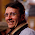## Saturday, May 14, 2011

### Jump or not jump

>Given an array of integers of size N, each element represents the max number of jumps can make forward. What is the minimum number of element selections to reach the end of the array starting from the first element. Please note that you need to land exactly on the end of the array and not after that point. Obviously you always have a strategy to reach the end in N moves by always avoiding to jump and deciding to move to the next position but N is not necessarily the minimum.

Example: arr = 1, 3, 5, 8, 9, 2, 6, 7, 6, 8, 9
Here the min # of selections is : 3 with the sequence 1-> 3 -> 8 ->9
First element is 1, so can only go to 3.
Second element is 3, so can make at most 3 jumps: eg to 5 (2+1 goes to 3 where you can jump to 5) or 8 (2+2 goes to 4 where you can jump to 8) or 9 (2+3 goes to 5 where you can jump to 9)
If an element is 0, then cannot make any jumps

There is a simple quadratic solution with Dynamic Programming, but I was not able to get any linear solution. Is it possible?

1.I think you can do n log n time in linear space. Hint: RMQ

2.I think you can just read the array left-to-right, keeping the interval of positions reachable after k (but not after k-1) steps as state.

k=0. You start at position 0, i.e. anywhere in [0,0].

k=1. From [0,0] you can go to anywhere in [0,1] (by choosing the 1 at 0). Removing the original interval puts us anywhere in [1,1] after step 1.

k=2. From [1,1] you can go to [1,4] (by choosing the 3 at 1). Removing the original interval puts us anywhere in [2,4] after step 2.

k=3. From [2,4] you can go to [2,7] (by choosing the 5 at 2), to [3,11] (by choosing the 8 at 3), or to [4,13] (by choosing the 9 at 4). We compute the union in linear time - it is [2,13]. Removing the original interval puts us anywhere in [5,13] after step 3.

Now, the current interval contains the end position (10), so the answer is k=3.

3.@w not sure about the logic, not all the positions in [5,13] are reachable in k=3 steps. Am i missing something?

@ot Range minimum queries how? Also, i was looking for a o(n) solution if possible.

4.@w, aha! gotcha while running at the gym

5.To continue on ot: if I understood well the problem for every position p it is the best move to jump on field q where q + a_q is maximum for all reachable fields of p. Then use range query to find q. But isn't the cost of RMQ ? http://en.wikipedia.org/wiki/Range_Minimum_Query